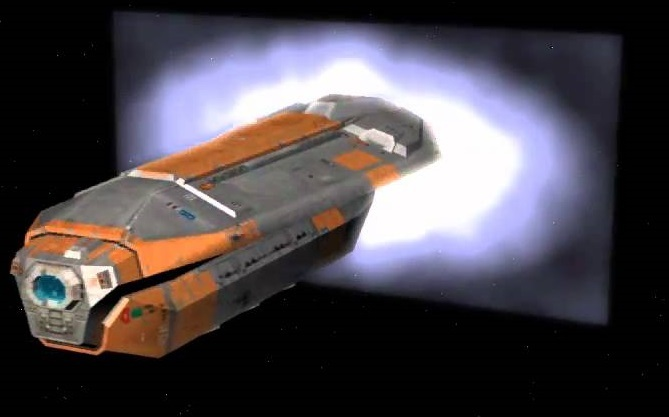# Is this possible using a simple shader?

• Have a quad which is invisible
• Compute the Quad Plane of it and pass it to Shader params ?
• The object is constantly moving towards the quad
• Maybe in pixel shader before drawing the pixel test it against the plane ?
• I have limited knowledge in shader, any hint or idea what I should implement is very much appreciated ^_^y

I have limited shader knowledge too, but I’m positive it can be done. I think it’d be “as easy as” getting the 3D position in the pixel shader and applying the formula to know which side of a plane that position is. Negative discard, positive shade.

However if the normal of the cutting plane is not pointing to the screen, you’ll see an ugly hole in your model because you’re clipping pixels, not adding geometry. (Imagine you cut a shoebox in half. If you look from the front it’s ok, but if you look from the back you see the hole)

1 Like

Thanks for replying I’ll see if I can grasp all of that : -D I think your correct if I can filter the pixel if beyond the plane draw it otherwise discard it… Ill check if can put that in code : - D

I really want to do something like thisThanks bro ^_^Y

• You position the object at the same place as the plane in the same direction

• You then find out the normal of the plane (IE. The direction that the plane is facing)

public static Vector3 calcNormal(Vector3 a, Vector3 b, Vector3 c)
{
Vector3 v1 = b - a;
Vector3 v2 = c - a;
return Vector3.Cross(v1, v2);
}

• Then find out half the width of the object, multiply it by the negative of the normal and then set that as the position (So that it ends up on the other side of the plane)

• Then add the normal to the object’s position to move it parallel to the plane (And therefore through the plane)

1 Like

Use the old hack:

http://xboxforums.create.msdn.com/forums/t/56786.aspx

MG doesn’t include clipplane stuff (it differs between platforms slightly). Shaderwise you’ll need a macro for OpenGL as it only has a fixed `discard` and not a function `clip(float)` (which clips when < 0).

1 Like

Thanks for all the input guyz… appreciated much… I’ll get back and post what will happen ^_^y

I think that example on the xna site was more for like fog, for what your showing i think you will need a couple extra steps.

You would need to pass to the shader, 4x vector3’s that describe each of the two planes.
These would denote

1. A positional point that lies on each plane.
2. The planes normalized surface direction.
(you will need two as shown in your image)

On the shader you would then have to perform the following steps. (in the same coordinate system)

1. Subtract the current pixel position from both passed plane positions (planePos - pixPos) you gave to the shader and normalize those results
2. Then dot each of those results to the normal directions you passed into the shader for each plane.
3. Check the resulting sign of both dot products to ensure they are within the boundrys.

if both resulting signs are positive you draw the pixel.
if they are not you clip the pixel.

Or you can probably just multiply the results of the dots and pass it to clip.
It’s basically the same as a polyhedron to point collision test you will of course need a seperate shader to draw the quad image as shown in the second image.

If this isn’t clear post back and ill see if i cant write out a basic shader for the steps.

1 Like

It’s a plane-clipping. It’s using `XYZ = normal, W = signed distance along normal` instead of `position, normal` (so just an XYZW instead of XYZ+XYZ). If you don’t care about anything origin relative (like a texture projection) then the origin is unnecessary.

I do the exact same thing for a quick-preview in a tool (just adjusted for plane-bounded volumes) before commiting to doing the heavier work CPU-side.

1 Like